Next: Largest square enclosed in Up: Possible parameters values for Previous: Possible parameters values for   Contents

Approximation of the set of solutions

Let consider the parameters space i.e. adimensional space where each of the dimension corresponds to one of theparameters. A point in this space corresponds to a unique value for all the parameters and therefore to a specific polynomial. In the parameters space there are possibly a set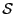of regions such that for any point in the region(s) the corresponding polynomial has all its root within the given interval. The purpose of the following procedure is to determine an approximation of. This approximation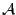will be constituted of a set ofdimensional boxes which are guaranteed to be included inand that will be written in a file. During the calculation the boxes whose width is lower than a given threshold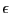and for which the algorithm has been unable to determine if they are fully enclosed inwill be neglected. A possible index for measuring the quality of the approximationis the ratio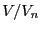between the total volume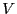of the boxes written into the file over the total volume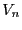of the boxes that have been neglected as the volume ofis lower or equal to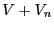.

The procedure is:

int ALIAS_Min_Max_EigenValues_Area(int Degree,int Nb_Parameter,
int Has_Interval,
INTERVAL_VECTOR (* TheCoeff)(INTERVAL_VECTOR &),
INTERVAL_VECTOR (* TheCoeffCentered)(INTERVAL_VECTOR &,double),
int Nb_Constraints,INTEGER_VECTOR &Type_Eq,
int (* TheMatrix)(INTERVAL_VECTOR &, INTERVAL_MATRIX &),
int Has_Matrix,
INTERVAL_VECTOR (* IntervalFunction)(int,int,INTERVAL_VECTOR &),
INTERVAL & TheDomain,INTERVAL_VECTOR & TheDomain_Parameter,
int Nb_Points,int Use_Solve,int rand,int Strong,int Iteration,
double Accuracy_Variable,double Accuracy,double AccuracyM,double AccuracyB,
double *Volume_Result,double *Volume_Neglected,double Seuil,
char *FileName,int Has_Input,char *File_Input,
int (* Solve_Poly)(double *, int *,double *),int RealRoot,
INTERVAL_VECTOR (* Evaluate_Complex)(int,int,INTERVAL_VECTOR &),
int (* Simp_Proc)(INTERVAL_VECTOR &))
where the arguments are similar to the one of the previous procedure except for:
• TheCoeffCentered: a procedure that returns the coefficients in x of the polynomial P(x+a). Takes as argument the parameters vector and a
• AccuracyB: the thresholdfor the maximal width of the neglected boxes
• Volume_Result: the total volume of the boxes that have been determined to be enclosed in the regions
• Volume_Neglected: the total volume of the boxes that have been neglected
• Seuil: the interval [Seuil, Seuil] defines the allowed range for the real roots of the polynomial
• FileName: the name of the file in which will be written the boxes that are included in the regions
• Has_Input, File_Input: the purpose of these variables is to allow an incremental improvement of the approximation. Indeed after a first run with a giventhe quality index may be not satisfactory. It is possible to improve it by decreasing the value offor a second run but it means that the boxes that have been determined to be enclosed in the region during the first run will be considered again, thereby leading to a loss of efficiency. These arguments allow a better control. During the first run if Has_Input has been set to 1 the neglected boxes will be stored in the file File_Input. During the second run (and the subsequent run if needed) Has_Input will be set to 2 and the set of boxes to be considered by the algorithm will be read from the file File_Input. During this type of run the neglected boxes will still be written in the file, allowing another run of the algorithm if needed. Hence the total volume of the boxes enclosed in the region will be the sum of the Volume_Result while the total volume of the neglected boxes will be the obtained during the last run of the algorithm. If Has_Input is set to 3 the neglected boxes will not be saved in a file.
• Strong: if 1 we use a secondary algorithm to determine if for a given box all the polynomial roots are in the range
• RealRoot: 0 if we are considering only real roots, 1 for the real part of the roots, 2 if we consider polynomial whose roots are all real and 3 if we consider polynomial with at least one real root
• Evaluate_Complex(i1,i2,X: let P be the polynomial and U=P(Seuil_First_Sol+I b), V=P(Seuil_First_Sol+I b). Let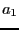be the real part of U,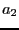the complex part of U,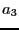the real part of V and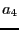the complex part of V. The procedure will return in its interval vector the value of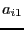to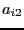. X is a Nb_Parameter+1 interval vector, the last one being the value of b

This procedure returns the number of boxes written in the result file or a negative number if the calculation has failed. The possible negative return code are:

• -2, -3: errors on the bounds for the roots
• -10: Iteration is lower than 10
• other values: the procedure that compute the minima and maximal real rots in a box has failedNext: Largest square enclosed in Up: Possible parameters values for Previous: Possible parameters values for   Contents
Jean-Pierre Merlet 2012-12-20